Rigid Body Dynamics Library
LoopConstraint Class Reference

Implements a rigid kinematic loop (or body-to-body) constraints as described in Ch. 8 of Featherstone's Rigid Body Dynamics Algorithms book. More...

#include <Constraint_Loop.h>Inheritance diagram for LoopConstraint:

## Public Member Functions

LoopConstraint ()

LoopConstraint (const unsigned int bodyIdPredecessor, const unsigned int bodyIdSuccessor, const Math::SpatialTransform &XPredecessor, const Math::SpatialTransform &XSuccessor, const Math::SpatialVector &constraintAxisInPredessor, bool enableBaumgarteStabilization=false, double stabilizationTimeConstant=0.1, const char *loopConstraintName=NULL, unsigned int userDefinedId=std::numeric_limits< unsigned int >::max(), bool positionLevelConstraint=true, bool velocityLevelConstraint=true)

void bind (const Model &model) override
Any local memory that has a dimension of N, where N is the length of QDot, is resized in this function.
More...

void calcConstraintJacobian (Model &model, const double time, const Math::VectorNd &Q, const Math::VectorNd &QDot, Math::MatrixNd &GSysUpd, ConstraintCache &cache, bool updateKinematics=false) override
In this function the matrix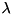of this contraint is inserted into the system constraint Jacobian(GSysOutput). The submatrixbegins at rowInSystem, has sizeOfConstraint number of rows, and fills the full number of columns in G. More...

void calcGamma (Model &model, const double time, const Math::VectorNd &Q, const Math::VectorNd &QDot, const Math::MatrixNd &GSys, Math::VectorNd &gammaSysUpd, ConstraintCache &cache, bool updateKinematics=false) override
In this function the vector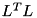of this constraint is inserted into the right-hand-side vector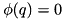of the system. The vectorbegins at rowInSystem, and has sizeOfConstraint rows. More...

void calcPositionError (Model &model, const double time, const Math::VectorNd &Q, Math::VectorNd &errSysUpd, ConstraintCache &cache, bool updateKinematics=false) override
In this function the sub vectorof this constraint is inserted into the position error vector of the system. If the constraint is velocity-level constraint or higher (noted in the boolean member variable positionConstraint) this should be set to zero. The vectorbegins at rowInSystem, and has sizeOfConstraint rows. More...

void calcVelocityError (Model &model, const double time, const Math::VectorNd &Q, const Math::VectorNd &QDot, const Math::MatrixNd &GSys, Math::VectorNd &derrSysUpd, ConstraintCache &cache, bool updateKinematics=false) override
In this function the sub vector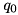of this constraint is inserted into the position error vector of the system. If the constraint is an acceleration-level constraint (noted in the boolean member variable velocityConstraint) this sub vector should be zero. The vectorbegins at rowInSystem, and has sizeOfConstraint rows. More...

void calcConstraintForces (Model &model, const double time, const Math::VectorNd &Q, const Math::VectorNd &QDot, const Math::MatrixNd &GSys, const Math::VectorNd &lagrangeMultipliersSys, std::vector< unsigned int > &constraintBodiesUpd, std::vector< Math::SpatialTransform > &constraintBodyFramesUpd, std::vector< Math::SpatialVector > &constraintForcesUpd, ConstraintCache &cache, bool resolveAllInRootFrame=false, bool updateKinematics=false) override
This function resolves the generalized forces this constraint applies to the system into the wrenches that are applied to the bodies that are involved in the constraint. More...

const std::vector< Math::SpatialVector > & getConstraintAxes ()

void appendConstraintAxis (const Math::SpatialVector &constraintAxis, bool positionLevelConstraint=true, bool velocityLevelConstraint=true)Public Member Functions inherited from Constraint
virtual void bind (const Model &model)=0
Any local memory that has a dimension of N, where N is the length of QDot, is resized in this function.
More...

virtual void calcConstraintJacobian (Model &model, const double time, const Math::VectorNd &Q, const Math::VectorNd &QDot, Math::MatrixNd &GSysOutput, ConstraintCache &cache, bool updateKinematics=false)=0
In this function the matrixof this contraint is inserted into the system constraint Jacobian(GSysOutput). The submatrixbegins at rowInSystem, has sizeOfConstraint number of rows, and fills the full number of columns in G. More...

virtual void calcGamma (Model &model, const double time, const Math::VectorNd &Q, const Math::VectorNd &QDot, const Math::MatrixNd &GSys, Math::VectorNd &gammaSysOutput, ConstraintCache &cache, bool updateKinematics=false)=0
In this function the vectorof this constraint is inserted into the right-hand-side vectorof the system. The vectorbegins at rowInSystem, and has sizeOfConstraint rows. More...

virtual void calcPositionError (Model &model, const double time, const Math::VectorNd &Q, Math::VectorNd &errSysOutput, ConstraintCache &cache, bool updateKinematics=false)=0
In this function the sub vectorof this constraint is inserted into the position error vector of the system. If the constraint is velocity-level constraint or higher (noted in the boolean member variable positionConstraint) this should be set to zero. The vectorbegins at rowInSystem, and has sizeOfConstraint rows. More...

virtual void calcVelocityError (Model &model, const double time, const Math::VectorNd &Q, const Math::VectorNd &QDot, const Math::MatrixNd &GSys, Math::VectorNd &derrSysOutput, ConstraintCache &cache, bool updateKinematics=false)=0
In this function the sub vectorof this constraint is inserted into the position error vector of the system. If the constraint is an acceleration-level constraint (noted in the boolean member variable velocityConstraint) this sub vector should be zero. The vectorbegins at rowInSystem, and has sizeOfConstraint rows. More...

virtual void calcConstraintForces (Model &model, const double time, const Math::VectorNd &Q, const Math::VectorNd &QDot, const Math::MatrixNd &GSys, const Math::VectorNd &LagrangeMultipliersSys, std::vector< unsigned int > &constraintBodiesOutput, std::vector< Math::SpatialTransform > &constraintBodyFramesOutput, std::vector< Math::SpatialVector > &constraintForcesOutput, ConstraintCache &cache, bool resolveAllInRootFrame=false, bool updateKinematics=false)=0
This function resolves the generalized forces this constraint applies to the system into the wrenches that are applied to the bodies that are involved in the constraint. More...

virtual ~Constraint ()

Constraint ()

Constraint (const char *nameOfConstraint, unsigned int typeOfConstraint, unsigned int sizeOfConstraint, unsigned int userDefinedIdNumber)

void addToConstraintSet (const unsigned int rowIndex)
This function is called by the functions in ConstraintSet that add a Constraint to the system. DO NOT TOUCH THIS. More...

unsigned int getUserDefinedId ()

void setUserDefinedId (unsigned int userDefinedId)

void getConstraintJacobian (const Math::MatrixNd &GSys, Math::MatrixNd &GConstraint)

void getGamma (const Math::VectorNd &gammaSys, Math::VectorNd &gammaConstraint)

void getPositionError (const Math::VectorNd &errSys, Math::VectorNd &errConstraint)

void getVelocityError (const Math::VectorNd &derrSys, Math::VectorNd &derrConstraint)

void getBaumgarteStabilizationParameters (Math::Vector2d &bgParamsUpd)

void getBaumgarteStabilizationForces (const Math::VectorNd &errPos, const Math::VectorNd &errVel, Math::VectorNd &baumgarteForces)

void addInBaumgarteStabilizationForces (const Math::VectorNd &errPosSys, const Math::VectorNd &errVelSys, Math::VectorNd &gammaSysOutput)

unsigned int getConstraintType ()

unsigned int getConstraintSize ()

unsigned int getConstraintIndex ()

void setBaumgarteTimeConstant (double tStab)
Calculates and sets the Baumgarte stabilization coefficients as a function of tStab, the approximate time-constant of stabilization. More...

void setEnableBaumgarteStabilization (bool flagEnableBaumgarteStabilization)

bool isBaumgarteStabilizationEnabled ()

void enableConstraintErrorFromPositionLevel (unsigned int constraintSubIndex)
: Will set the vectors positionConstraint and velocityConstraint to be consistent with a constraint that is described from the position-level up. More...

void enableConstraintErrorFromVelocityLevel (unsigned int constraintSubIndex)
: Will set the vectors positionConstraint and velocityConstraint to be consistent with a constraint that is described from the velocity-level up: position errors will be set to zero while velocity errors will be computed. More...

void enableConstraintErrorFromAccelerationLevel (unsigned int constraintSubIndex)
: Will set the vectors positionConstraint and velocityConstraint to be consistent with a constraint that is described from the acceleration-level: both position and velocity errors will be set to zero. More...

void enableConstraintErrorFromPositionLevel ()
: Will set the all elements in positionConstraint and velocityConstraint to be consistent with a constraint that is described from the position-level up. More...

void enableConstraintErrorFromVelocityLevel ()
: Will set the all elements in positionConstraint and velocityConstraint to be consistent with a constraint that is described from the velocity-level up. More...

void enableConstraintErrorFromAccelerationLevel ()
: Will set the all elements in positionConstraint and velocityConstraint to be consistent with a constraint that is described from the acceleration-level up. More...

bool getPositionLevelError (unsigned int constraintSubIndex)
Returns the boolean value that determines whether or not position-level errors are computed for this sub-index into this constraint. More...

bool getVelocityLevelError (unsigned int constraintSubIndex)
Returns the boolean value that determines whether or not velocity-level errors are computed for this sub-index into this constraint. More...

const char * getName ()

const std::vector< unsigned int > & getBodyIds ()

const std::vector< Math::SpatialTransform > & getBodyFrames ()

## Private Attributes

std::vector< Math::SpatialVectorT
Vector of constraint axis resolved in the predecessor frame. More...

double dblA
A local working double. More...Protected Attributes inherited from Constraint
std::string name
A user defined name which is unique to this constraint set. More...

unsigned int id
A user defined id which is unique to this constraint set. More...

unsigned int typeOfConstraint
The type of this constraint. More...

unsigned int sizeOfConstraint
The number of rows that this constraint adds to G. More...

unsigned int rowInSystem
The first row in G that corresponds to this constraint. More...

std::vector< unsigned int > bodyIds
The index of the predecessor body in the vector of bodies in Model. More...

std::vector< Math::SpatialTransformbodyFrames
Transform from the frame of the predecessor body to the constraint frame. More...

Math::Vector2d baumgarteParameters

bool baumgarteEnabled
A flag which enables or disables Baumgarte stabilization. More...

std::vector< bool > positionConstraint

std::vector< bool > velocityConstraint

## Detailed Description

Implements a rigid kinematic loop (or body-to-body) constraints as described in Ch. 8 of Featherstone's Rigid Body Dynamics Algorithms book.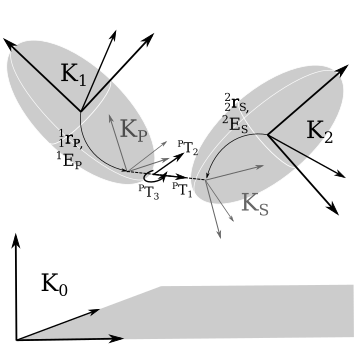A loop constraint restricts the movement of a point p located on the predecessor body relative to point s on a successor body in the directions T_i.

For details on this constraint please do read this documentation. However note that a typical user of RBDL should not need to use any of the functions described in this class but instead should use the functions that are defined in ConstraintSet (AddContactConstraint, calcForces, calcPositionError, calcVelocityError, calcBaumgarteStabilizationForces, etc) when working with this constraint. For those interested in all of the details of this constraint and how it works please refer to the source code for this class, and its base class.

A LoopConstraint will zero the acceleration between body-fixed points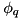and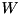in the spatial directions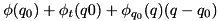. The user specifiesandby passing in the spatial transform from the origin of the predecessor body to the predecessor frame (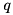,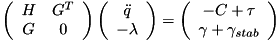) and likewise for the successor body to the successor frame (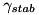,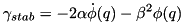). The spatial directions that the constraint is applied are specified in the vectorwhich is resolved in the coordinates of the predecessor frame.

As with any other constraint this position-level constraint is index reduced and applied at the acceleration level. This constraint will only be satisfied at the position-level and velocity-level if, prior to enabling the constraint, the constraint equations are satisified at the position and velocity levels. When assembling the constraint the functions CalcAssemblyQ and CalcAssemblyQDot can be used to satisfy this constraint at the position and velocity levels.

During the process of integration numerical error may accumulate. To prevent this error from growing Baumgarte stabilization can be enabled using the function setEnableBaumgarteStabilization provided in the base class. Numerical drift is usually small with this constraint becauseis body fixed. When this constraint is used in an optimal control problem Baumgarte stabilization may also be used to guide the solver to physically valid model configurations. Without stabilization the solver may introduce large constraint errors which will otherwise not be reduced. By default Baumgarte stabilization is not enabled.

By default this constraint does have its position-level and velocity-level errors defined. This has the consequence that CalcAssemblyQ will update the position of the model to satisfy these constraints, as will
CalcAssemblyQDot at the velocity level. In addition, Baumgarte stabilization will apply forces to the model in response to constraint errors at both the position and velocity level.

Definition at line 72 of file Constraint_Loop.h.

## ◆ LoopConstraint() [1/2]

 LoopConstraint ( )

Definition at line 26 of file Constraint_Loop.cc.

## ◆ LoopConstraint() [2/2]

 LoopConstraint ( const unsigned int bodyIdPredecessor, const unsigned int bodyIdSuccessor, const Math::SpatialTransform & XPredecessor, const Math::SpatialTransform & XSuccessor, const Math::SpatialVector & constraintAxisInPredessor, bool enableBaumgarteStabilization = false, double stabilizationTimeConstant = 0.1, const char * loopConstraintName = NULL, unsigned int userDefinedId = std::numeric_limits::max(), bool positionLevelConstraint = true, bool velocityLevelConstraint = true )
Parameters
 bodyIdPredecessor the identifier of the predecessor body bodyIdSuccessor the identifier of the successor body XPredecessor a spatial transform localizing the constrained frames on the predecessor body, expressed with respect to the predecessor body frame. Note the position vector should be the r_BPB (from the body's origin, to the predessor frame, in the coordinates of the body's frame) and E_BP (the rotation matrix that will rotate vectors from the coordinates of the P frame to the coordinates of the body's frame). XSuccessor a spatial transform localizing the constrained frames on the successor body, expressed with respect to the successor body frame. Note the position vector should be the r_BSB (from the body's origin, to the successor frame, in the coordinates of the body's frame) and E_BS (the rotation matrix that will rotate vectors from the coordinates of the S frame to the coordinates of the body's frame). constraintAxisInPredessor a spatial vector, resolved in the frame of the predecessor frame, indicating the axis along which the constraint acts. enableBaumgarteStabilization (optional, default false) setting this flag to true will modify the right hand side of the acceleration equation with a penaltiy term that is proportional to the constraint error scaled by a constant. stabilizationTimeConstant (optional, defaults to 0.1 sec) this value scales the strength of Baumgarte stabilization so that the settling time of the error is proportional the value given here. name a human readable name (optional, default: NULL). Set this field to a unique name (within this ConstraintSet) so that the function GetConstraintIndex can find it. userDefinedId a user defined id (optional, defaults to max()). Set this field to a unique number (within this ConstraintSet) so that the function GetConstraintIndex can find it. positionLevelConstraint (optional, defaults to true to be consistent with the original implementation): When set to true, position errors will be computed for this constraint. This has the consequence that the function CalcAssemblyQ will assemble this constraint at the position level. In addition if Baumgarte stabilization is enabled, stabilization forces will be applied as a function of the position error. velocityLevelConstraint (optional, defaults to true to be consistent with the original implementation) : This flag controls whether or not velocity errors are computed for this constraint. When velocity errors are computed they are used by CalcAssemblyQDot (to assemble this constraint at the velocity level) and by Baumgarte stabilization (if it is enabled) to modify the right hand side of the acceleration equation with a penalty term proportional to error.

Definition at line 31 of file Constraint_Loop.cc.

## ◆ appendConstraintAxis()

 void appendConstraintAxis ( const Math::SpatialVector & constraintAxis, bool positionLevelConstraint = true, bool velocityLevelConstraint = true )
Parameters
 constraintAxis to append. This constraintAxis must have a norm of 1 and be normal to the existing constraintAxis positionLevelConstraint if true position errors will be evaluated for this constraint entry. velocityLevelConstraint if true velocity errors will be evalulated for this constraint entry.

Definition at line 351 of file Constraint_Loop.cc.

## ◆ bind()

 void bind ( const Model & model )
overridevirtual

Any local memory that has a dimension of N, where N is the length of QDot, is resized in this function.

Parameters
 model a reference to the multibody model. Use the model's fields of qdot_size to size local memory (if any) that depends on the model.

Implements Constraint.

Definition at line 73 of file Constraint_Loop.cc.

## ◆ calcConstraintForces()

 void calcConstraintForces ( Model & model, const double time, const Math::VectorNd & Q, const Math::VectorNd & QDot, const Math::MatrixNd & GSys, const Math::VectorNd & LagrangeMultipliersSys, std::vector< unsigned int > & constraintBodiesOutput, std::vector< Math::SpatialTransform > & constraintBodyFramesOutput, std::vector< Math::SpatialVector > & constraintForcesOutput, ConstraintCache & cache, bool resolveAllInRootFrame = false, bool updateKinematics = false )
overridevirtual

This function resolves the generalized forces this constraint applies to the system into the wrenches that are applied to the bodies that are involved in the constraint.

Parameters
 model a reference to the multibody model. time the time which is included so that rheonomic constraints might be included (in the future). Q the vector of generalized positions. QDot the vector of generalized velocities. GSys a reference to the constraint Jacobian for the entire system. Ifis needed in this function do not re-evaluate it but instead extract it from the system: this constraint's sub-matrix begins at rowInSystem, has sizeOfConstraint rows, and the full number of columns of GSys. LagrangeMultipliersSys the vector of Lagrange multipliers for the entire system. The Lagrange multipliers for this constraint begin at rowInSystem and has sizeOfConstraint elements. constraintBodiesOutput contains the indices of the bodies that contain the local frames listed in constraintBodyFramesOutput. If resolveAllInRootFrame is true, all of these indicies should be set to 0: in this case all of the wrenches are resolved in the root frame. constraintBodyFramesOutput contains the local transformation from the origin of each body frame listed in constraintBodiesOutput to the frame in which the constraint wrench is applied. Note the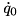frame is located on thebody. If resolveAllInRootFrame is true, all of these frames have their origins resolved in the global frame and their orientation set to match that tof the global frame. constraintForcesOutput contains the wrenches that the constraint applies to the local frames listed in constraintBodyFramesOutput. Note theforce is resolved in theframe.If resolveAllInRootFrame is true, this wrench should be rotated into the coordinates of the root frame. cache a ConstraintCache object which contains ample pre-allocated memory that can be used to reduce the memory footprint of each Constraint implementation. resolveAllInRootFrame When this parameter is - false: the wrenches are resolved into their local frames. - true: the wrenches are resolved into coordinates of the global frame  updateKinematics setting this flag to true will cause all calls to kinematic dependent functions to be updated using the generalized coordinates passed into this function.

Implements Constraint.

Definition at line 269 of file Constraint_Loop.cc.

## ◆ calcConstraintJacobian()

 void calcConstraintJacobian ( Model & model, const double time, const Math::VectorNd & Q, const Math::VectorNd & QDot, Math::MatrixNd & GSysOutput, ConstraintCache & cache, bool updateKinematics = false )
overridevirtual

In this function the matrixof this contraint is inserted into the system constraint Jacobian(GSysOutput). The submatrixbegins at rowInSystem, has sizeOfConstraint number of rows, and fills the full number of columns in G.

Parameters
 model a reference to the multibody model. time the time which is included so that rheonomic constraints might be included (in the future). Q the vector of generalized positions. QDot the vector of generalized velocities. GSysOutput a reference to the constraint Jacobian for the entire system. Insert the G sub-matrix for this constraint beginning and rowInSystem, with a length sizeOfConstraint rows, and a width of the full number of columns in GSysOutput. cache a ConstraintCache object which contains ample pre-allocated memory that can be used to reduce the memory footprint of each Constraint implementation. updateKinematics setting this flag to true will cause all calls to kinematic dependent functions to be updated using the generalized coordinates passed into this function.

Implements Constraint.

Definition at line 83 of file Constraint_Loop.cc.

## ◆ calcGamma()

 void calcGamma ( Model & model, const double time, const Math::VectorNd & Q, const Math::VectorNd & QDot, const Math::MatrixNd & GSys, Math::VectorNd & gammaSysOutput, ConstraintCache & cache, bool updateKinematics = false )
overridevirtual

In this function the vectorof this constraint is inserted into the right-hand-side vectorof the system. The vectorbegins at rowInSystem, and has sizeOfConstraint rows.

Parameters
 model a reference to the multibody model. time the time which is included so that rheonomic constraints might be included (in the future). Q the vector of generalized positions. QDot the vector of generalized velocities. GSys a reference to the constraint Jacobian for the entire system. Ifis needed in this function do not re-evaluate it but instead extract it from the system. gammaSysOutput the system's gamma vector. Insert the gamma sub-vector for this constraint beginning and rowInSystem and with a length of sizeOfConstraint rows. cache a ConstraintCache object which contains ample pre-allocated memory that can be used to reduce the memory footprint of each Constraint implementation. updateKinematics setting this flag to true will cause all calls to kinematic dependent functions to be updated using the generalized coordinates passed into this function.

Implements Constraint.

Definition at line 122 of file Constraint_Loop.cc.

## ◆ calcPositionError()

 void calcPositionError ( Model & model, const double time, const Math::VectorNd & Q, Math::VectorNd & errSysOutput, ConstraintCache & cache, bool updateKinematics = false )
overridevirtual

In this function the sub vectorof this constraint is inserted into the position error vector of the system. If the constraint is velocity-level constraint or higher (noted in the boolean member variable positionConstraint) this should be set to zero. The vectorbegins at rowInSystem, and has sizeOfConstraint rows.

Parameters
 model a reference to the multibody model time the time which is included so that rheonomic constraints might be included (in the future). Q the vector of generalized positions. errSysOutput the system's constraint position error vector. Insert the position error sub-vector for this constraint beginning and rowInSystem and with a length of sizeOfConstraint rows. cache a ConstraintCache object which contains ample pre-allocated memory that can be used to reduce the memory footprint of each Constraint implementation. updateKinematics setting this flag to true will cause all calls to kinematic dependent functions to be updated using the generalized coordinates passed into this function.

Implements Constraint.

Definition at line 176 of file Constraint_Loop.cc.

## ◆ calcVelocityError()

 void calcVelocityError ( Model & model, const double time, const Math::VectorNd & Q, const Math::VectorNd & QDot, const Math::MatrixNd & GSys, Math::VectorNd & derrSysOutput, ConstraintCache & cache, bool updateKinematics = false )
overridevirtual

In this function the sub vectorof this constraint is inserted into the position error vector of the system. If the constraint is an acceleration-level constraint (noted in the boolean member variable velocityConstraint) this sub vector should be zero. The vectorbegins at rowInSystem, and has sizeOfConstraint rows.

Parameters
 model a reference to the multibody model. time the time which is included so that rheonomic constraints might be included (in the future). Q the vector of generalized positions. QDot the vector of generalized velocities. GSys a reference to the constraint Jacobian for the entire system. Ifis needed in this function do not re-evaluate it but instead extract it from the system: this constraint's sub-matrix begins at rowInSystem, has sizeOfConstraint rows, and the full number of columns of GSys. derrSysOutput the system's constraint velocity error vector. Insert the velocity error sub-vector for this constraint beginning and rowInSystem and with a length of sizeOfConstraint rows. cache a ConstraintCache object which contains ample pre-allocated memory that can be used to reduce the memory footprint of each Constraint implementation. updateKinematics setting this flag to true will cause all calls to kinematic dependent functions to be updated using the generalized coordinates passed into this function.

Implements Constraint.

Definition at line 238 of file Constraint_Loop.cc.

## ◆ getConstraintAxes()

 const std::vector< Math::SpatialVector > & getConstraintAxes ( )
inline
Returns
the vector of constraint axes which are resolved in the coordinate system of the predecessor frame

Definition at line 215 of file Constraint_Loop.h.

## ◆ dblA

 double dblA
private

A local working double.

Definition at line 236 of file Constraint_Loop.h.

## ◆ T

 std::vector< Math::SpatialVector > T
private

Vector of constraint axis resolved in the predecessor frame.

Definition at line 234 of file Constraint_Loop.h.

The documentation for this class was generated from the following files: